111
Searching - Code Monk
Search Algorithms
CodeMonk

A. Introduction

Searching is one of the most fundamental problems in Computer Science. It is the process of finding a particular item in a collection of items. Typically, a search answers whether the item is present in the collection or not. For simplification for all our examples, we will be taking an array as the collection.

B. Linear Search (Sequential Search)

Linear Search is the simplest method to solve the searching problem. It finds an item in a collection by looking for it from the beginning and looks for it till the end. Basically, it checks each item in the sequence until the desired element is found before all the elements of the collection are exhausted.

Code for the linear search is:

bool linearSearch (int A[], int length, int item) {
for (int i = 0 ; i < length ; ++i)
if (item == A[i])
return true;                // Item is found in array A
}


Consider an example. A = {1, 9, 2, 4, 6, 3, 7, 5, 8, 0} and suppose we need to find 3 in the given array. Linear search starts from the beginning and checks if it matches with the item we are searching.

In the example the item = 3 and length = 10.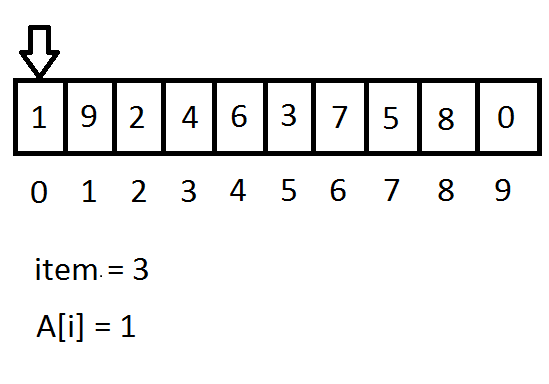For i = 0, A[i] = 1 and item is not equal to A[i].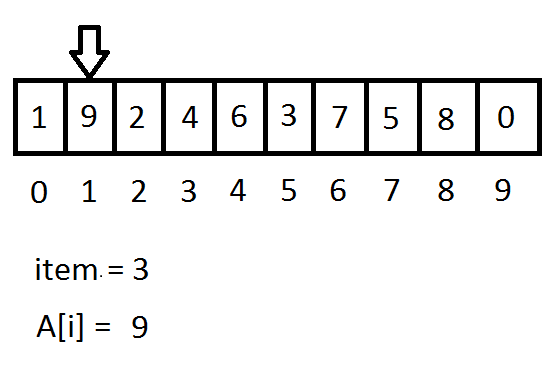For i = 1, A[i] = 9 and item is not equal to A[i].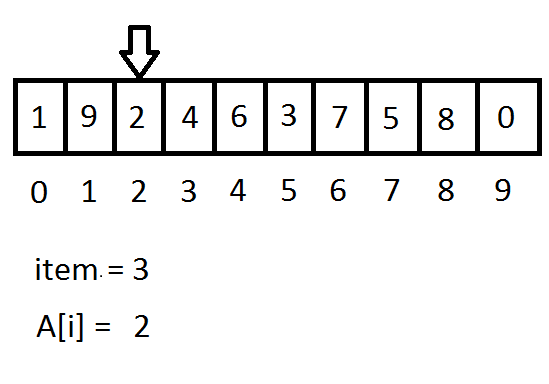For i = 2, A[i] = 2 and item is not equal to A[i].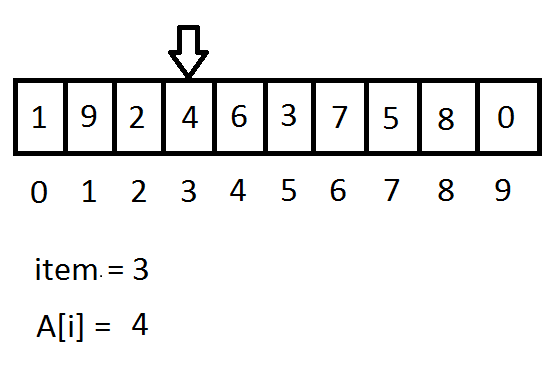For i = 3, A[i] = 4 and item is not equal to A[i].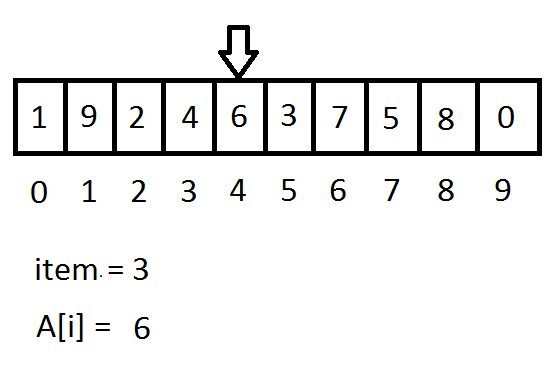For i = 4, A[i] = 6 and item is not equal to A[i].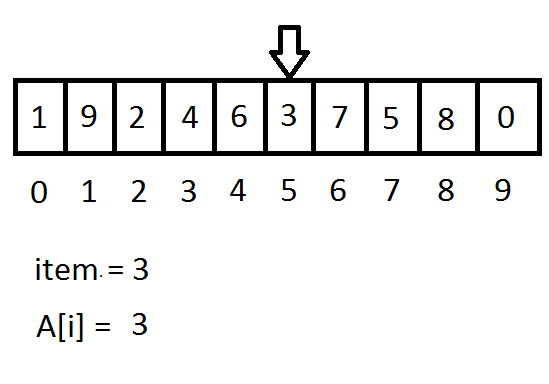For i = 5, A[i] = 3 and item is equal to A[i]. So the linearSearch() function will return true showing that the item is found.

If the item = 10, linearSearch() function will iterate over all the elements in the array A and return false (showing that the item is not found), because the ‘if’ condition will never be true.

Now, let us consider the modified search where we need to return the position, where the item is found. To solve this modified search problem, we just need to change 2 lines in the linearSearch() function.

int linearSearch ( int A[ ], int length, int item) {
for (int i = 0; i < length ; ++i)
if (item == A[i])
return i;            //Returning the index of the element found.
}


In the above codes -1 denotes that the item is not found.

Note: If there are duplicate elements, the above code will return the first occurrence of the item.

Exercise: Modify linearSearch() function such that it will return the last occurrence of the item, if the item is in the array A, otherwise return -1. (Hint: Store the position of the last found occurrence of the item and update it as soon as the item occurs again)

The time complexity of linear search is O(size of the array).

C. Binary Search

Binary Search is a Divide and Conquer algorithm. In Divide and Conquer, we reduce a larger problem into smaller subproblems recursively until the problem is small enough that the solution is trivial.

A condition for Binary Search is that the array should be a sorted array. If the array is sorted in ascending order, Binary Search compares the item with the middle element of the array. If the item matches, then it returns true. Otherwise, if the item is less than the middle element then it will recurse on the sub-array to the left of the middle element or if the item is greater then it will recurse on the sub-array to the right of the middle element. So, at each step the size of array will be reduced to half. After repeating this recursion, it will eventually be left with a single element.

Pseudocode for Binary Search is

Algorithm binarySearch(A, left, right, item) {
if left is less than or equal to right then :
mid = (left + right) / 2
if A[mid] is equal to item then return true
else if item is less than A[mid] then recurse on the left subarray
else if item is greater than A[mid] recurse on the right subarray
else
return false
}


Note: Array has to be sorted for Binary Search to work.

Implementation of Binary search is

1. Recursive Binary Search

bool binarySearchRecur(int A[], int left, int right, int item)
{
if (left <= right)
{
int mid = left + (right - left) / 2;            // finding middle index
if (A[mid] == item)
return true;                    // item found
else if (item < A[mid])
{
// recurse on the left sub-array
return binarySearchRecur(A, left, mid-1, item);
}
else
{
// recurse on the right sub-array
return binarySearchRecur(A, mid+1, right, item);
}
}
else
}


2. Iterative Binary Search

bool binarySearchIter(int A[], int length, int item)
{
int left = 0, right = length - 1, mid;
while (left <= right)
{
mid = left + (right - left) / 2;        // finding middle index
if (A[mid] == item)
return true;                // item found
else if (item < A[mid])
right = mid - 1;            // recurse on left sub-array
else
left = mid + 1;             // recurse on right sub-array
}
}


Consider an example. Let array A = {0, 1, 2, 3, 4, 5, 6, 7, 8, 9} and we need to find 3 in array A. So item = 3 and length = 10. Let us consider the Iterative Binary Search.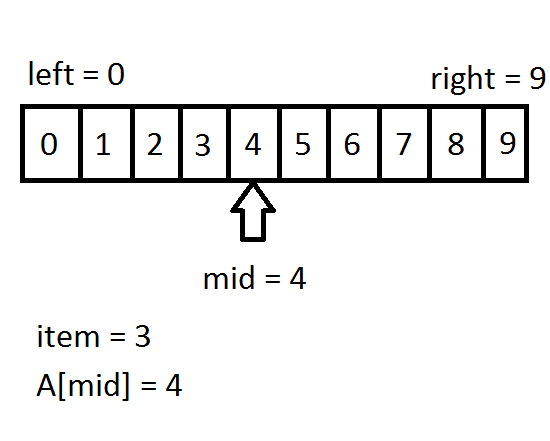Initially,
left = 0
right = length – 1 = 10 – 1 = 9
In 1st iteration,
mid = left + (right – left) / 2 = 0 + (9 – 0) / 2 = 4
A[mid] ( = 4) is greater than item. Since the array A is sorted we can say that the item must be in the left sub-array.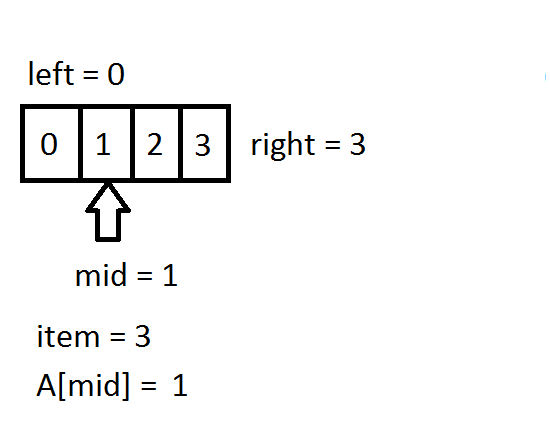So,
left = 0
right = mid – 1 = 4 – 1 = 3
In 2nd iteration,
mid = left + (right – left) / 2 = 0 + (3 – 0) / 2 = 1
A[mid] ( = 1) is smaller than item. Since the array A is sorted we can say that the item must be in the right sub-array.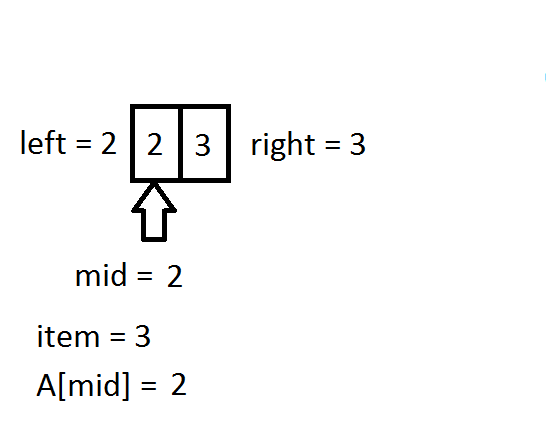So,
left = mid + 1 = 1 + 1 = 2
right = 3
In 3rd iteration,
mid = left + (right – left) / 2 = 2 + (3 – 2) / 2 = 2
A[mid] ( = 2) is smaller than item. Since the array A is sorted we can say that the item must be in the right sub-array.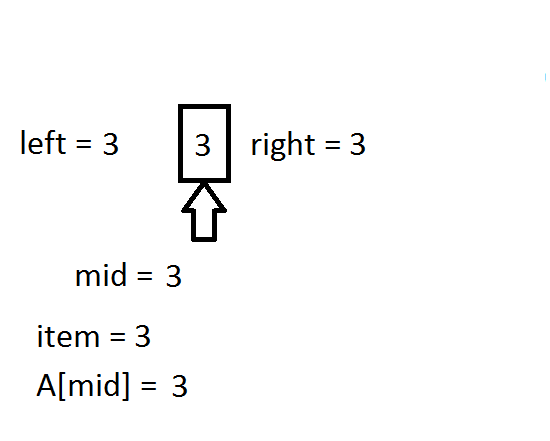So,
left = mid + 1 = 2 + 1 = 3
right = 3
In 4th iteration,
mid = left + (right – left) / 2 = 3 + (3 – 3) / 2 = 3
A[mid] ( = 3) is equal to the item, so the binarySearchIter() function will return true and the item is found.

Time complexity of both binarySearchRecur() and binarySearchIter() is O(logN) where N is the size of the array. This is because after each recursion or each iteration the size of problem is reduced into half.

Some more examples:

Question 1:
Given a sorted array A with possible duplicate elements and you need to find the first occurrence of a given input item (assuming the element is present in the array).

Solution:
If the item is less than or equal to the middle element we will recurse to the left sub-array, otherwise we will recurse to the right sub-array.

int firstOccurrence(int A[], int left, int right, int item) {
int mid;
while (right - left > 1) {
mid = left + (right - left) / 2;
if (A[mid] >= item)
right = mid;
else
left = mid;
}
return right;
}


Question 2: Given a sorted array A with possible duplicate elements and you need to find the last occurrence of a given input item (assuming the element is present in the array).

Solution: If the item is greater than or equal to the middle element we will recurse to the right sub-array, otherwise we will recurse to the left sub-array.

int lastOccurrence(int A[], int left, int right, int item) {
int mid;
while (right - left > 1) {
mid = left + (right - left) / 2;
if (A[mid] <= item)
left = mid;
else
right = mid;
}
return left;
}


Question 3: Given a sorted array A with possible duplicate elements and you need to find the number of occurrences of a given input item (assuming the element is present in the array).

Solution: If we can find the first and last occurrence of the item in the array A then number of occurrences of the item will be number of elements from first occurrence to the last occurrence.

int numberOfOccurrences(int A[], int size, int item) {
// Note the boundary parameters
int left = firstOccurrence(A, -1, size - 1, item);
int right = lastOccurrence(A, 0, size, item);
if (A[left] == item && A[right] == item)            // If the item is in the array A
return (right - left + 1);
else                                                            // If the item is not in the array A
return 0;
}


Binary Search is a simple and a powerful tool for you to keep in your toolbox. But, a problem as easy as searching for a particular element, will rarely be faced by you in your programming career - not directly, at least. So, the task is to identify and reduce a given problem into a problem which can thus be solved by using binary search. This can be done if we reduce the original problem into a Yes/No Problem.

Let me try to clear the grounds by giving an example. Suppose there is a problem in which we have a monotonically increasing function f (i.e. f(i) < f(j) if i < j). We need to find a smallest x such that f(x) > 0.

Can we apply binary search in this problem? Yes, we can. But firstly, we need to reduce the problem into a simpler one. Instead of “finding smallest x such that f(x) > 0”, we will find the answer of “Is there any x such that f(x) > 0 ?”. If we can somehow find the smallest x for which answer to the second question is Yes then that x will be the answer of our original problem. Now, we can apply binary search to find the smallest x such that f(x) > 0.

Initially, we have a range [low, high]. We will check the middle element of this range i.e mid = (low + high) / 2. If f(mid) <= 0 then recurse on the range [mid+1, high] i.e on the right side of the mid element, otherwise recurse on the range [low, mid] i.e on the left side of the mid element. Repeat this algorithm and you will end up with a single value and that will be the answer.

For advanced users who are comfortable with STL: There are a couple of C++ STL functions which are associated closely with Binary Search, and can be used, to ease out a lot of things:

1. binary_search(): Return true if the item in equal to any element in the range passed as parameter.
2. lower_bound(): Returns an iterator to the first element which is greater than or equal to the item.
3. upper_bound(): Returns an iterator to the first element which is greater than the item.
4. equal_range(): Returns a pair of iterators whose first element is equal to the lower_bound() and second element is equal to the upper_bound().

All these four STL functions are a part of the header in C++.

Example:

#include <bits/stdc++.h>
#include <algorithm>
using namespace std;
int main() {
int A[] = {5, 2, 6, 2, 2, 1, 1, 6, 3, 2};
vector <int> v(A, A + 10);            // v =  5, 2, 6, 2, 2, 1, 1, 6, 3, 2
sort (v.begin(), v.end());                // v =  1, 1, 2, 2, 2, 2, 3, 5, 6, 6
vector <int>::iterator it;
if(binary_search(v.begin(), v.end(), 2))
cout << "2 is in the array" << endl;
else
cout << "2 is not in the array" << endl;
it = lower_bound(v.begin(), v.end(), 2);
cout << "lower_bound: " << (it - v.begin()) << endl;
it = upper_bound(v.begin(), v.end(), 2);
cout << "upper_bound: " << (it - v.begin()) << endl;
pair < vector <int>::iterator, vector <int>::iterator > p;
p = equal_range(v.begin(), v.end(), 2);
cout << "equal_range First: " << (p.first - v.begin()) << endl;
cout << "equal_range Second: " <<  (p.second - v.begin()) << endl;
return 0;
}


Output:
2 is in the array
lower_bound: 2
upper_bound: 6
equal_range First: 2
equal_range Second: 6

Practice Problems: